#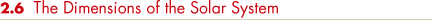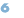Kepler's laws allow us to construct a scale model of the solar system, with the correct shapes and relative sizes of all the planetary orbits, but they do not tell us the actual size of any orbit. We can express the distance to each planet only in terms of the distance from Earth to the Sun. Why is this? Because Kepler's triangulation measurements all used a portion of Earth's orbit as a baseline, distances could be expressed only relative to the size of that orbit, which was not itself determined. Thus our model of the solar system would be analogous to a road map of the United States showing the relative positions of cities and towns but lacking the all-important scale marker indicating distances in kilometers or miles. For example, we would know that Kansas City is about three times more distant from New York than it is from Chicago, but we would not know the actual mileage between any two points on the map.

If we could somehow determine the value of the astronomical unit—in kilometers, say—we would be able to add the vital scale marker to our map of the solar system and compute the exact distances between the Sun and each of the planets. We might propose using triangulation to measure the distance from Earth to the Sun directly. However, we would find it impossible to measure the Sun's parallax using Earth's diameter as a baseline. The Sun is too bright, too big, and too fuzzy for us to distinguish any apparent displacement relative to a field of distant stars. To measure the Sun's distance from Earth, we must resort to some other method.

Before the middle of the twentieth century, the most accurate measurements of the astronomical unit were made using triangulation on the planets Mercury and Venus during their rare transits of the Sun—that is, during the brief periods when those planets passed directly between the Sun and Earth (as shown for the case of Mercury in Figure 2.15). Because the time at which a transit occurs can be determined with great precision, astronomers can use this information to make very accurate measurements of a planet's position in the sky. They can then use simple geometry to compute the distance to the planet by combining observations made from different locations on Earth, as discussed earlier in Chapter 1.(Sec. 1.5) For example, the parallax of Venus at closest approach to Earth, as seen from two diametrically opposite points on Earth (separated by about 13,000 km), is about 1 arc minute—at the limit of naked-eye capabilities but easily measurable telescopically. This parallax represents a distance of 45 million km.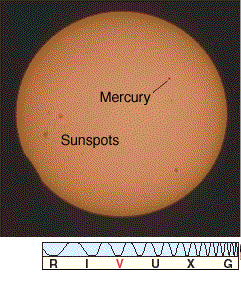Figure 2.15 A solar transit of Mercury. Such transits happen only about once per decade, because Mercury's orbit does not quite coincide with the plane of the ecliptic. Transits of Venus are even rarer, occurring only about twice per century.

Knowing the distance to Venus, we can compute the magnitude of the astronomical unit. Figure 2.16 is an idealized diagram of the Sun—Earth—Venus orbital geometry. The planetary orbits are drawn as circles here, but in reality they are slight ellipses. This is a subtle difference, and we can correct for it using detailed knowledge of orbital motions. Assuming for the sake of simplicity that the orbits are perfect circles, we see from the figure that the distance from Earth to Venus at closest approach is approximately 0.3 A.U. Knowing that 0.3 A.U. is 45,000,000 km makes determining 1 A.U. straightforward—the answer is 45,000,000/0.3, or 150,000,000 km.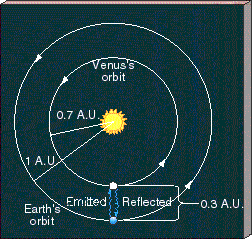Figure 2.16 Simplified geometry of the orbits of Earth and Venus as they move around the Sun. The wavy lines represent the paths along which radar signals might be transmitted toward Venus and received back at Earth at the moment when Venus is at its minimum distance from Earth. Because the radius of Earth's orbit is 1 A.U. and that of Venus is about 0.7 A.U., we know that this distance is 0.3 A.U. Thus, radar measurements allow us to determine the astronomical unit in kilometers.

The modern method for deriving the absolute scale of the solar system uses radar rather than triangulation. The word radar is an acronym for radio detection and ranging. In this technique, radio waves are transmitted toward an astronomical body, such as a planet. (We cannot use radar ranging to measure the distance to the Sun directly because radio signals are absorbed at the solar surface and are not reflected to Earth.) The returning echo indicates the body's direction and range, or distance, in absolute terms—that is, in kilometers rather than in astronomical units. Multiplying the round-trip travel time of the radar signal (the time elapsed between transmission of the signal and reception of the echo) by the speed of light (300,000 km/s, which is also the speed of radio waves), we obtain twice the distance to the target planet.

Venus, whose orbit periodically brings it closest to Earth, is the most common target for radar ranging. The round-trip travel time (for example, at closest approach, as indicated by the wavy lines on Figure 2.16) can be measured with high precision—in fact, well enough to determine the planet's distance to an accuracy of about 1 km. In this way, the astronomical unit is now known to be 149,597,870 km. We will use the rounded-off value of 1.5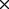108 km in this text. (For more on the use of scientific notation to represent very large or very small numbers, see Appendix 1.)

Having determined the value of the astronomical unit, we can reexpress the sizes of the other planetary orbits in terms of more familiar units, such as miles or kilometers. The entire scale of the solar system can then be calibrated to high precision.